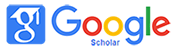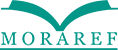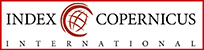### HARD WORK AND PROBLEM SOLVING BASED ON KRULIK-RUDNICK'S HEURISTIC THEORY ON PROJECT BASED LEARNING WITH OQALE APPROACH OF 5th GRADE ELEMENTARY SCHOOL STUDENTS

Tri Suryaningsih

#### Abstract

The study aimed to describe the state of early poblem solving, the effectiveness of learning, and discription the application of the learning to had work character and problem solving mathematics students. The research is the kind of research concurerent embedded. Technic the data with using interviews, observation, and tests. Technic data analysis using analysis discriptif and statistical tests influence regression, the appeal, and test an increase in the gain. The results of the study showed that the initial conditions problem solving students average be low; effectiveness of indicated by the average capability problem solving mathematical students experiment has reached KKM that is above 74,5%, the ability problem solving mathematical class experiment better than class control, hard work and skill problem solving mathematical students have had a positive impact of the ability of mathematical problem soling students, with big the influence of 88,7%, increased capacity problem solving mathematical class experiment higher than class control; the results of a description of this research is improving hard work students; skills literacy mathematics; and ability problem solving mathematical stdents with a score of the gain 0,59; 0,66; 0,70.

#### Keywords

Oqale; Hard Work; Krulik-Rudnick; Problem Solving; Project Based Learning

#### Full Text:

PDF

DOI: http://dx.doi.org/10.32934/jmie.v3i1.101

### Refbacks

• There are currently no refbacks.